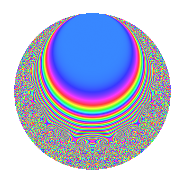# Properties

 Label 294.6.aLevel $294$ Weight $6$ Character orbit 294.a Rep. character $\chi_{294}(1,\cdot)$ Character field $\Q$ Dimension $33$ Newform subspaces $23$ Sturm bound $336$ Trace bound $11$

# Related objects

## Defining parameters

 Level: $$N$$ $$=$$ $$294 = 2 \cdot 3 \cdot 7^{2}$$ Weight: $$k$$ $$=$$ $$6$$ Character orbit: $$[\chi]$$ $$=$$ 294.a (trivial) Character field: $$\Q$$ Newform subspaces: $$23$$ Sturm bound: $$336$$ Trace bound: $$11$$ Distinguishing $$T_p$$: $$5$$, $$11$$

## Dimensions

The following table gives the dimensions of various subspaces of $$M_{6}(\Gamma_0(294))$$.

Total New Old
Modular forms 296 33 263
Cusp forms 264 33 231
Eisenstein series 32 0 32

The following table gives the dimensions of the cuspidal new subspaces with specified eigenvalues for the Atkin-Lehner operators and the Fricke involution.

$$2$$$$3$$$$7$$FrickeDim.
$$+$$$$+$$$$+$$$$+$$$$3$$
$$+$$$$+$$$$-$$$$-$$$$5$$
$$+$$$$-$$$$+$$$$-$$$$4$$
$$+$$$$-$$$$-$$$$+$$$$4$$
$$-$$$$+$$$$+$$$$-$$$$4$$
$$-$$$$+$$$$-$$$$+$$$$4$$
$$-$$$$-$$$$+$$$$+$$$$3$$
$$-$$$$-$$$$-$$$$-$$$$6$$
Plus space$$+$$$$14$$
Minus space$$-$$$$19$$

## Trace form

 $$33q + 4q^{2} + 9q^{3} + 528q^{4} + 22q^{5} + 36q^{6} + 64q^{8} + 2673q^{9} + O(q^{10})$$ $$33q + 4q^{2} + 9q^{3} + 528q^{4} + 22q^{5} + 36q^{6} + 64q^{8} + 2673q^{9} - 360q^{10} - 652q^{11} + 144q^{12} + 694q^{13} + 198q^{15} + 8448q^{16} - 1054q^{17} + 324q^{18} + 3124q^{19} + 352q^{20} - 1696q^{22} + 5424q^{23} + 576q^{24} + 24427q^{25} - 4392q^{26} + 729q^{27} + 1262q^{29} - 5976q^{30} + 616q^{31} + 1024q^{32} + 180q^{33} + 13704q^{34} + 42768q^{36} - 31658q^{37} + 2192q^{38} - 10134q^{39} - 5760q^{40} - 20742q^{41} + 3684q^{43} - 10432q^{44} + 1782q^{45} + 14080q^{46} + 13776q^{47} + 2304q^{48} - 89124q^{50} + 11718q^{51} + 11104q^{52} - 63538q^{53} + 2916q^{54} - 69672q^{55} + 80100q^{57} + 2440q^{58} - 80900q^{59} + 3168q^{60} + 86566q^{61} + 106848q^{62} + 135168q^{64} + 146500q^{65} + 37008q^{66} + 123956q^{67} - 16864q^{68} - 88920q^{69} + 161888q^{71} + 5184q^{72} + 105274q^{73} + 349528q^{74} + 48807q^{75} + 49984q^{76} - 48024q^{78} + 212748q^{79} + 5632q^{80} + 216513q^{81} + 13800q^{82} + 62612q^{83} + 158524q^{85} - 172368q^{86} + 188982q^{87} - 27136q^{88} - 121398q^{89} - 29160q^{90} + 86784q^{92} + 235656q^{93} + 129024q^{94} + 302848q^{95} + 9216q^{96} - 163646q^{97} - 52812q^{99} + O(q^{100})$$

## Decomposition of $$S_{6}^{\mathrm{new}}(\Gamma_0(294))$$ into newform subspaces

Label Dim. $$A$$ Field CM Traces A-L signs $q$-expansion
$$a_2$$ $$a_3$$ $$a_5$$ $$a_7$$ 2 3 7
294.6.a.a $$1$$ $$47.153$$ $$\Q$$ None $$-4$$ $$-9$$ $$-26$$ $$0$$ $$+$$ $$+$$ $$-$$ $$q-4q^{2}-9q^{3}+2^{4}q^{4}-26q^{5}+6^{2}q^{6}+\cdots$$
294.6.a.b $$1$$ $$47.153$$ $$\Q$$ None $$-4$$ $$-9$$ $$-26$$ $$0$$ $$+$$ $$+$$ $$-$$ $$q-4q^{2}-9q^{3}+2^{4}q^{4}-26q^{5}+6^{2}q^{6}+\cdots$$
294.6.a.c $$1$$ $$47.153$$ $$\Q$$ None $$-4$$ $$-9$$ $$72$$ $$0$$ $$+$$ $$+$$ $$-$$ $$q-4q^{2}-9q^{3}+2^{4}q^{4}+72q^{5}+6^{2}q^{6}+\cdots$$
294.6.a.d $$1$$ $$47.153$$ $$\Q$$ None $$-4$$ $$-9$$ $$86$$ $$0$$ $$+$$ $$+$$ $$+$$ $$q-4q^{2}-9q^{3}+2^{4}q^{4}+86q^{5}+6^{2}q^{6}+\cdots$$
294.6.a.e $$1$$ $$47.153$$ $$\Q$$ None $$-4$$ $$9$$ $$-86$$ $$0$$ $$+$$ $$-$$ $$-$$ $$q-4q^{2}+9q^{3}+2^{4}q^{4}-86q^{5}-6^{2}q^{6}+\cdots$$
294.6.a.f $$1$$ $$47.153$$ $$\Q$$ None $$-4$$ $$9$$ $$-44$$ $$0$$ $$+$$ $$-$$ $$-$$ $$q-4q^{2}+9q^{3}+2^{4}q^{4}-44q^{5}-6^{2}q^{6}+\cdots$$
294.6.a.g $$1$$ $$47.153$$ $$\Q$$ None $$-4$$ $$9$$ $$26$$ $$0$$ $$+$$ $$-$$ $$-$$ $$q-4q^{2}+9q^{3}+2^{4}q^{4}+26q^{5}-6^{2}q^{6}+\cdots$$
294.6.a.h $$1$$ $$47.153$$ $$\Q$$ None $$-4$$ $$9$$ $$54$$ $$0$$ $$+$$ $$-$$ $$-$$ $$q-4q^{2}+9q^{3}+2^{4}q^{4}+54q^{5}-6^{2}q^{6}+\cdots$$
294.6.a.i $$1$$ $$47.153$$ $$\Q$$ None $$4$$ $$-9$$ $$-24$$ $$0$$ $$-$$ $$+$$ $$-$$ $$q+4q^{2}-9q^{3}+2^{4}q^{4}-24q^{5}-6^{2}q^{6}+\cdots$$
294.6.a.j $$1$$ $$47.153$$ $$\Q$$ None $$4$$ $$-9$$ $$6$$ $$0$$ $$-$$ $$+$$ $$-$$ $$q+4q^{2}-9q^{3}+2^{4}q^{4}+6q^{5}-6^{2}q^{6}+\cdots$$
294.6.a.k $$1$$ $$47.153$$ $$\Q$$ None $$4$$ $$9$$ $$-76$$ $$0$$ $$-$$ $$-$$ $$-$$ $$q+4q^{2}+9q^{3}+2^{4}q^{4}-76q^{5}+6^{2}q^{6}+\cdots$$
294.6.a.l $$1$$ $$47.153$$ $$\Q$$ None $$4$$ $$9$$ $$-6$$ $$0$$ $$-$$ $$-$$ $$+$$ $$q+4q^{2}+9q^{3}+2^{4}q^{4}-6q^{5}+6^{2}q^{6}+\cdots$$
294.6.a.m $$1$$ $$47.153$$ $$\Q$$ None $$4$$ $$9$$ $$66$$ $$0$$ $$-$$ $$-$$ $$-$$ $$q+4q^{2}+9q^{3}+2^{4}q^{4}+66q^{5}+6^{2}q^{6}+\cdots$$
294.6.a.n $$2$$ $$47.153$$ $$\Q(\sqrt{2})$$ None $$-8$$ $$-18$$ $$-108$$ $$0$$ $$+$$ $$+$$ $$+$$ $$q-4q^{2}-9q^{3}+2^{4}q^{4}+(-54+5\beta )q^{5}+\cdots$$
294.6.a.o $$2$$ $$47.153$$ $$\Q(\sqrt{505})$$ None $$-8$$ $$-18$$ $$-17$$ $$0$$ $$+$$ $$+$$ $$-$$ $$q-4q^{2}-9q^{3}+2^{4}q^{4}+(-9-\beta )q^{5}+\cdots$$
294.6.a.p $$2$$ $$47.153$$ $$\Q(\sqrt{505})$$ None $$-8$$ $$18$$ $$17$$ $$0$$ $$+$$ $$-$$ $$+$$ $$q-4q^{2}+9q^{3}+2^{4}q^{4}+(9+\beta )q^{5}+\cdots$$
294.6.a.q $$2$$ $$47.153$$ $$\Q(\sqrt{2})$$ None $$-8$$ $$18$$ $$108$$ $$0$$ $$+$$ $$-$$ $$+$$ $$q-4q^{2}+9q^{3}+2^{4}q^{4}+(54+5\beta )q^{5}+\cdots$$
294.6.a.r $$2$$ $$47.153$$ $$\Q(\sqrt{9601})$$ None $$8$$ $$-18$$ $$-53$$ $$0$$ $$-$$ $$+$$ $$+$$ $$q+4q^{2}-9q^{3}+2^{4}q^{4}+(-26-\beta )q^{5}+\cdots$$
294.6.a.s $$2$$ $$47.153$$ $$\Q(\sqrt{4705})$$ None $$8$$ $$-18$$ $$-18$$ $$0$$ $$-$$ $$+$$ $$-$$ $$q+4q^{2}-9q^{3}+2^{4}q^{4}+(-9-\beta )q^{5}+\cdots$$
294.6.a.t $$2$$ $$47.153$$ $$\Q(\sqrt{2})$$ None $$8$$ $$-18$$ $$108$$ $$0$$ $$-$$ $$+$$ $$+$$ $$q+4q^{2}-9q^{3}+2^{4}q^{4}+(54+5\beta )q^{5}+\cdots$$
294.6.a.u $$2$$ $$47.153$$ $$\Q(\sqrt{2})$$ None $$8$$ $$18$$ $$-108$$ $$0$$ $$-$$ $$-$$ $$+$$ $$q+4q^{2}+9q^{3}+2^{4}q^{4}+(-54+5\beta )q^{5}+\cdots$$
294.6.a.v $$2$$ $$47.153$$ $$\Q(\sqrt{4705})$$ None $$8$$ $$18$$ $$18$$ $$0$$ $$-$$ $$-$$ $$-$$ $$q+4q^{2}+9q^{3}+2^{4}q^{4}+(9-\beta )q^{5}+\cdots$$
294.6.a.w $$2$$ $$47.153$$ $$\Q(\sqrt{9601})$$ None $$8$$ $$18$$ $$53$$ $$0$$ $$-$$ $$-$$ $$-$$ $$q+4q^{2}+9q^{3}+2^{4}q^{4}+(3^{3}-\beta )q^{5}+\cdots$$

## Decomposition of $$S_{6}^{\mathrm{old}}(\Gamma_0(294))$$ into lower level spaces

$$S_{6}^{\mathrm{old}}(\Gamma_0(294)) \cong$$ $$S_{6}^{\mathrm{new}}(\Gamma_0(3))$$$$^{\oplus 6}$$$$\oplus$$$$S_{6}^{\mathrm{new}}(\Gamma_0(6))$$$$^{\oplus 3}$$$$\oplus$$$$S_{6}^{\mathrm{new}}(\Gamma_0(7))$$$$^{\oplus 8}$$$$\oplus$$$$S_{6}^{\mathrm{new}}(\Gamma_0(14))$$$$^{\oplus 4}$$$$\oplus$$$$S_{6}^{\mathrm{new}}(\Gamma_0(21))$$$$^{\oplus 4}$$$$\oplus$$$$S_{6}^{\mathrm{new}}(\Gamma_0(42))$$$$^{\oplus 2}$$$$\oplus$$$$S_{6}^{\mathrm{new}}(\Gamma_0(49))$$$$^{\oplus 4}$$$$\oplus$$$$S_{6}^{\mathrm{new}}(\Gamma_0(98))$$$$^{\oplus 2}$$$$\oplus$$$$S_{6}^{\mathrm{new}}(\Gamma_0(147))$$$$^{\oplus 2}$$